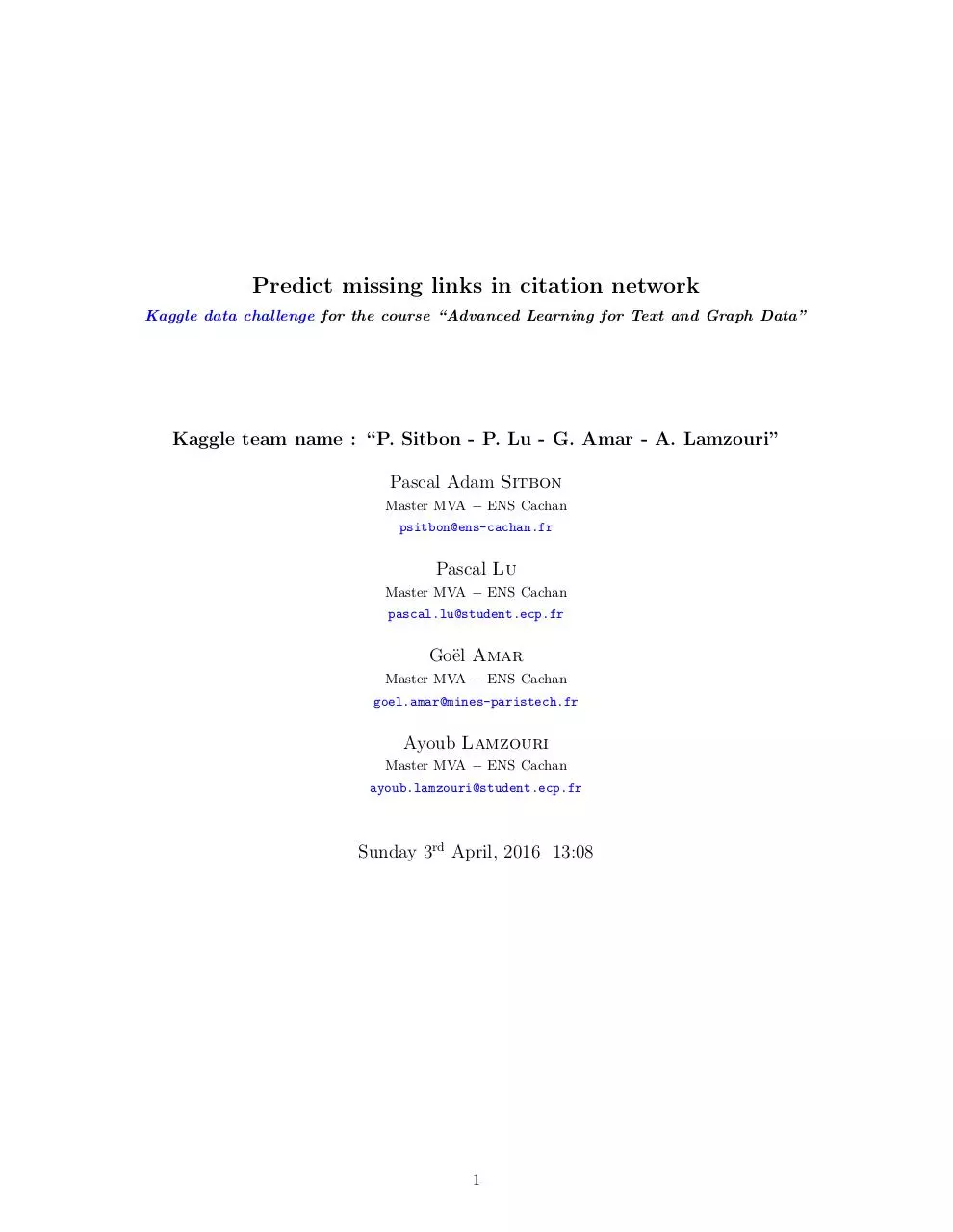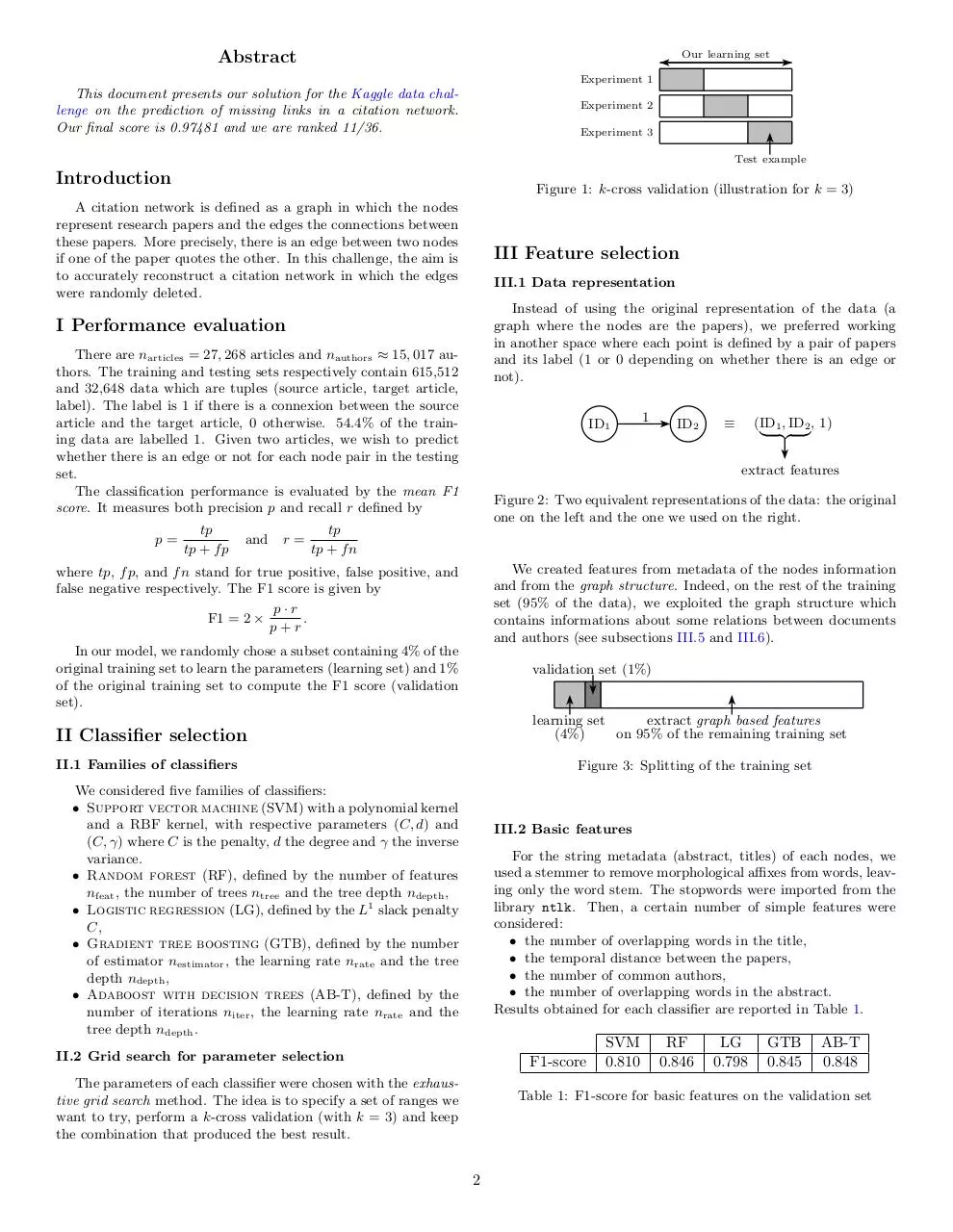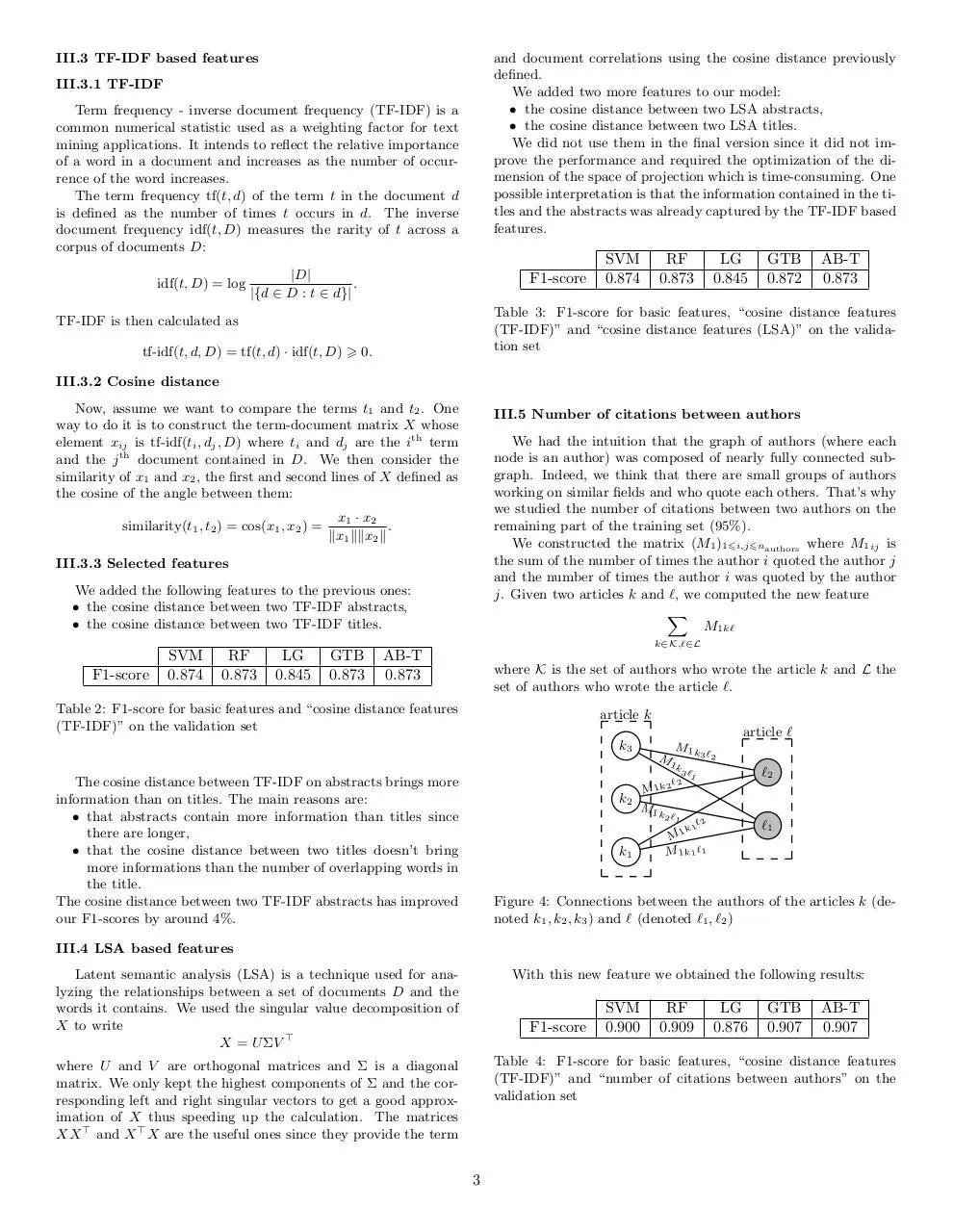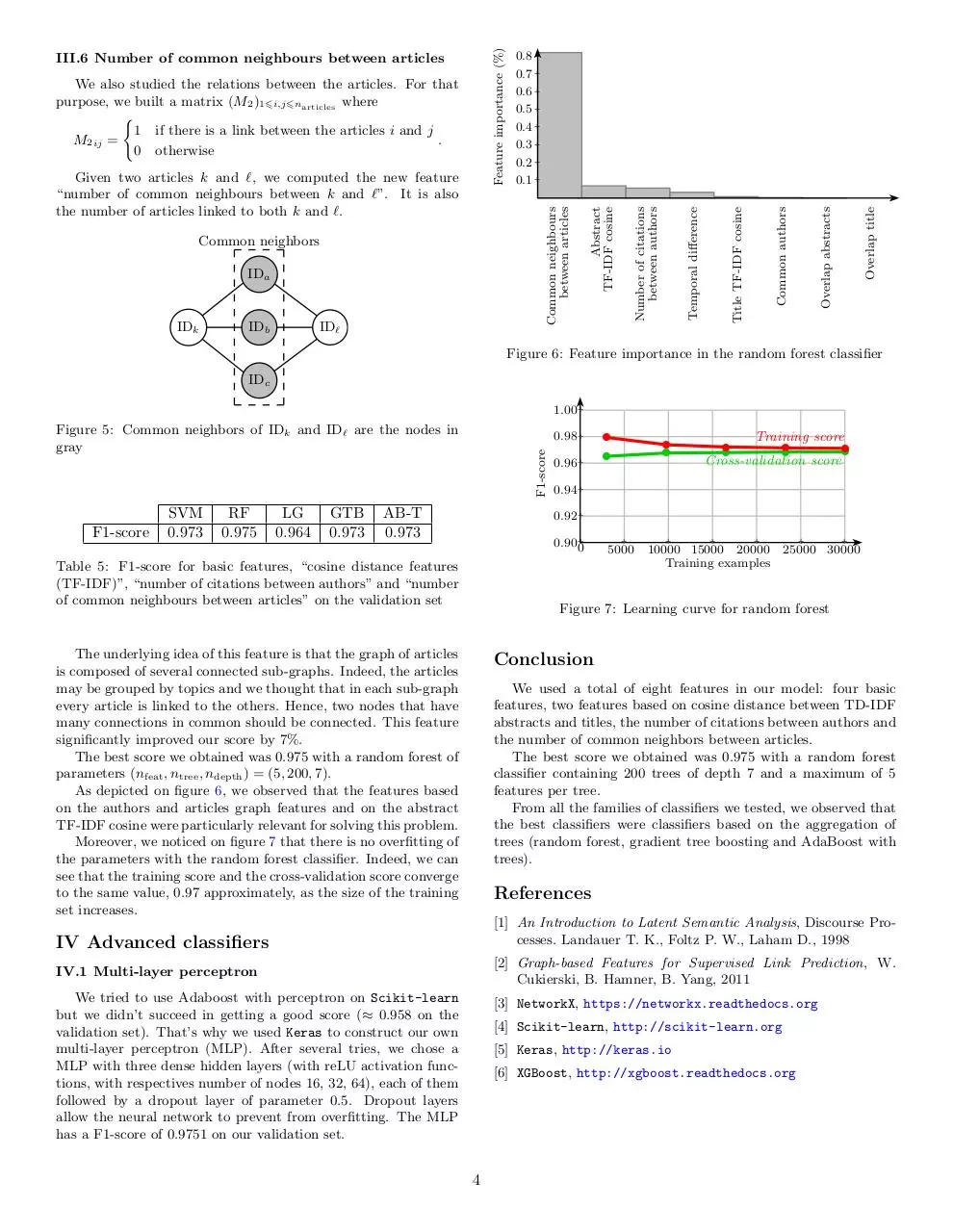# Graph (1) .pdf

### File information

This PDF 1.5 document has been generated by LaTeX with hyperref package / XeTeX 0.99992, and has been sent on pdf-archive.com on 19/01/2017 at 10:50, from IP address 185.127.x.x. The current document download page has been viewed 535 times.
File size: 95.66 KB (4 pages).
Privacy: public file

### Document preview

Predict missing links in citation network
Kaggle data challenge for the course “Advanced Learning for Text and Graph Data”

Kaggle team name : “P. Sitbon - P. Lu - G. Amar - A. Lamzouri”
Master MVA − ENS Cachan
psitbon@ens-cachan.fr

Pascal Lu
Master MVA − ENS Cachan
pascal.lu@student.ecp.fr

Go¨el Amar
Master MVA − ENS Cachan
goel.amar@mines-paristech.fr

Ayoub Lamzouri
Master MVA − ENS Cachan
ayoub.lamzouri@student.ecp.fr

Sunday 3rd April, 2016 13:08

1

Abstract

Our learning set
Experiment 1

This document presents our solution for the Kaggle data challenge on the prediction of missing links in a citation network.
Our final score is 0.97481 and we are ranked 11/36.

Experiment 2
Experiment 3
Test examples

Introduction

Figure 1: k-cross validation (illustration for k = 3)

A citation network is defined as a graph in which the nodes
represent research papers and the edges the connections between
these papers. More precisely, there is an edge between two nodes
if one of the paper quotes the other. In this challenge, the aim is
to accurately reconstruct a citation network in which the edges
were randomly deleted.

III Feature selection
III.1 Data representation
Instead of using the original representation of the data (a
graph where the nodes are the papers), we preferred working
in another space where each point is defined by a pair of papers
and its label (1 or 0 depending on whether there is an edge or
not).

I Performance evaluation
There are narticles = 27, 268 articles and nauthors ≈ 15, 017 authors. The training and testing sets respectively contain 615,512
and 32,648 data which are tuples (source article, target article,
label). The label is 1 if there is a connexion between the source
article and the target article, 0 otherwise. 54.4% of the training data are labelled 1. Given two articles, we wish to predict
whether there is an edge or not for each node pair in the testing
set.
The classification performance is evaluated by the mean F1
score. It measures both precision p and recall r defined by
p=

tp
tp + f p

and

r=

ID1

1

ID2

(ID1 , ID2 , 1)
| {z }
extract features

Figure 2: Two equivalent representations of the data: the original
one on the left and the one we used on the right.

tp
tp + f n
We created features from metadata of the nodes information
and from the graph structure. Indeed, on the rest of the training
set (95% of the data), we exploited the graph structure which
contains informations about some relations between documents
and authors (see subsections III.5 and III.6).

where tp, f p, and f n stand for true positive, false positive, and
false negative respectively. The F1 score is given by
p·r
F1 = 2 ×
.
p+r
In our model, we randomly chose a subset containing 4% of the
original training set to learn the parameters (learning set) and 1%
of the original training set to compute the F1 score (validation
set).

validation set (1%)

learning set
extract graph based features
(4%)
on 95% of the remaining training set

II Classifier selection
II.1 Families of classifiers

Figure 3: Splitting of the training set

We considered five families of classifiers:
• Support vector machine (SVM) with a polynomial kernel
and a RBF kernel, with respective parameters (C, d) and
(C, γ) where C is the penalty, d the degree and γ the inverse
variance.
• Random forest (RF), defined by the number of features
nfeat , the number of trees ntree and the tree depth ndepth ,
• Logistic regression (LG), defined by the L1 slack penalty
C,
• Gradient tree boosting (GTB), defined by the number
of estimator nestimator , the learning rate nrate and the tree
depth ndepth ,
• Adaboost with decision trees (AB-T), defined by the
number of iterations niter , the learning rate nrate and the
tree depth ndepth .

III.2 Basic features
For the string metadata (abstract, titles) of each nodes, we
used a stemmer to remove morphological aﬃxes from words, leaving only the word stem. The stopwords were imported from the
library ntlk. Then, a certain number of simple features were
considered:
• the number of overlapping words in the title,
• the temporal distance between the papers,
• the number of common authors,
• the number of overlapping words in the abstract.
Results obtained for each classifier are reported in Table 1.

II.2 Grid search for parameter selection

F1-score

The parameters of each classifier were chosen with the exhaustive grid search method. The idea is to specify a set of ranges we
want to try, perform a k-cross validation (with k = 3) and keep
the combination that produced the best result.

SVM
0.810

RF
0.846

LG
0.798

GTB
0.845

AB-T
0.848

Table 1: F1-score for basic features on the validation set

2

III.3 TF-IDF based features

and document correlations using the cosine distance previously
defined.
We added two more features to our model:
• the cosine distance between two LSA abstracts,
• the cosine distance between two LSA titles.
We did not use them in the final version since it did not improve the performance and required the optimization of the dimension of the space of projection which is time-consuming. One
possible interpretation is that the information contained in the titles and the abstracts was already captured by the TF-IDF based
features.

III.3.1 TF-IDF
Term frequency - inverse document frequency (TF-IDF) is a
common numerical statistic used as a weighting factor for text
mining applications. It intends to reflect the relative importance
of a word in a document and increases as the number of occurrence of the word increases.
The term frequency tf(t, d) of the term t in the document d
is defined as the number of times t occurs in d. The inverse
document frequency idf(t, D) measures the rarity of t across a
corpus of documents D:
|D|
idf(t, D) = log
.
|{d ∈ D : t ∈ d}|

F1-score

SVM
0.874

RF
0.873

LG
0.845

GTB
0.872

AB-T
0.873

Table 3: F1-score for basic features, “cosine distance features
(TF-IDF)” and “cosine distance features (LSA)” on the validation set

TF-IDF is then calculated as
tf-idf(t, d, D) = tf(t, d) · idf(t, D) ⩾ 0.
III.3.2 Cosine distance
Now, assume we want to compare the terms t1 and t2 . One
way to do it is to construct the term-document matrix X whose
element xij is tf-idf(ti , dj , D) where ti and dj are the ith term
and the j th document contained in D. We then consider the
similarity of x1 and x2 , the first and second lines of X defined as
the cosine of the angle between them:
similarity(t1 , t2 ) = cos(x1 , x2 ) =

III.5 Number of citations between authors
We had the intuition that the graph of authors (where each
node is an author) was composed of nearly fully connected subgraph. Indeed, we think that there are small groups of authors
working on similar fields and who quote each others. That’s why
we studied the number of citations between two authors on the
remaining part of the training set (95%).
We constructed the matrix (M1 )1⩽i,j⩽nauthors where M1ij is
the sum of the number of times the author i quoted the author j
and the number of times the author i was quoted by the author
j. Given two articles k and ℓ, we computed the new feature

M1kℓ

x1 · x2
.
∥x1 ∥∥x2 ∥

III.3.3 Selected features
We added the following features to the previous ones:
• the cosine distance between two TF-IDF abstracts,
• the cosine distance between two TF-IDF titles.

k∈K,ℓ∈L

F1-score

SVM
0.874

RF
0.873

LG
0.845

GTB
0.873

AB-T
0.873

where K is the set of authors who wrote the article k and L the
set of authors who wrote the article ℓ.

Table 2: F1-score for basic features and “cosine distance features
(TF-IDF)” on the validation set

article k
article ℓ
k3

M

M1 k

3 ℓ2

1k

ℓ2

3ℓ
1

The cosine distance between TF-IDF on abstracts brings more
information than on titles. The main reasons are:
there are longer,
• that the cosine distance between two titles doesn’t bring
the title.
The cosine distance between two TF-IDF abstracts has improved
our F1-scores by around 4%.

k2

ℓ2
M1 k2

M1 k

2 ℓ1

k1

ℓ2
k1

ℓ1

M1
M1k1 ℓ1

Figure 4: Connections between the authors of the articles k (denoted k1 , k2 , k3 ) and ℓ (denoted ℓ1 , ℓ2 )

III.4 LSA based features
With this new feature we obtained the following results:

Latent semantic analysis (LSA) is a technique used for analyzing the relationships between a set of documents D and the
words it contains. We used the singular value decomposition of
X to write
X = U ΣV ⊤

F1-score

SVM
0.900

RF
0.909

LG
0.876

GTB
0.907

AB-T
0.907

Table 4: F1-score for basic features, “cosine distance features
(TF-IDF)” and “number of citations between authors” on the
validation set

where U and V are orthogonal matrices and Σ is a diagonal
matrix. We only kept the highest components of Σ and the corresponding left and right singular vectors to get a good approximation of X thus speeding up the calculation. The matrices
XX ⊤ and X ⊤ X are the useful ones since they provide the term

3

IDk

IDb

IDℓ

Overlap title

Overlap abstracts

Common authors

IDa

Title TF-IDF cosine

Common neighbors

Temporal diﬀerence

Common neighbours
between articles

Given two articles k and ℓ, we computed the new feature
“number of common neighbours between k and ℓ”. It is also
the number of articles linked to both k and ℓ.

Number of citations
between authors

We also studied the relations between the articles. For that
purpose, we built a matrix (M2 )1⩽i,j⩽narticles where
{
1 if there is a link between the articles i and j
M2ij =
.
0 otherwise

0.8 +
0.7 +
0.6 +
0.5 +
0.4 +
0.3 +
0.2 +
0.1 +
Abstract
TF-IDF cosine

Feature importance (%)

III.6 Number of common neighbours between articles

Figure 6: Feature importance in the random forest classifier
IDc
1.00+

Figure 5: Common neighbors of IDk and IDℓ are the nodes in
gray

F1-score

SVM
0.973

RF
0.975

LG
0.964

GTB
0.973

b

F1-score

0.98+

AB-T
0.973

0.96+

b
b
b

Training score
b

b
b

b

bb

Cross-validation score

0.94+
0.92+
0.90+0

Table 5: F1-score for basic features, “cosine distance features
(TF-IDF)”, “number of citations between authors” and “number
of common neighbours between articles” on the validation set

+

5000

+

+

+

+

+

10000 15000 20000 25000 30000
Training examples

Figure 7: Learning curve for random forest

The underlying idea of this feature is that the graph of articles
is composed of several connected sub-graphs. Indeed, the articles
may be grouped by topics and we thought that in each sub-graph
every article is linked to the others. Hence, two nodes that have
many connections in common should be connected. This feature
significantly improved our score by 7%.
The best score we obtained was 0.975 with a random forest of
parameters (nfeat , ntree , ndepth ) = (5, 200, 7).
As depicted on figure 6, we observed that the features based
on the authors and articles graph features and on the abstract
TF-IDF cosine were particularly relevant for solving this problem.
Moreover, we noticed on figure 7 that there is no overfitting of
the parameters with the random forest classifier. Indeed, we can
see that the training score and the cross-validation score converge
to the same value, 0.97 approximately, as the size of the training
set increases.

Conclusion
We used a total of eight features in our model: four basic
features, two features based on cosine distance between TD-IDF
abstracts and titles, the number of citations between authors and
the number of common neighbors between articles.
The best score we obtained was 0.975 with a random forest
classifier containing 200 trees of depth 7 and a maximum of 5
features per tree.
From all the families of classifiers we tested, we observed that
the best classifiers were classifiers based on the aggregation of
trees).

References
 An Introduction to Latent Semantic Analysis, Discourse Processes. Landauer T. K., Foltz P. W., Laham D., 1998

 Graph-based Features for Supervised Link Prediction, W.
Cukierski, B. Hamner, B. Yang, 2011

IV.1 Multi-layer perceptron
We tried to use Adaboost with perceptron on Scikit-learn
but we didn’t succeed in getting a good score (≈ 0.958 on the
validation set). That’s why we used Keras to construct our own
multi-layer perceptron (MLP). After several tries, we chose a
MLP with three dense hidden layers (with reLU activation functions, with respectives number of nodes 16, 32, 64), each of them
followed by a dropout layer of parameter 0.5. Dropout layers
allow the neural network to prevent from overfitting. The MLP
has a F1-score of 0.9751 on our validation set.

 Scikit-learn, http://scikit-learn.org
 Keras, http://keras.io

4Graph (1).pdf (PDF, 95.66 KB)

### Share on social networks

#### HTML Code

Copy the following HTML code to share your document on a Website or Blog